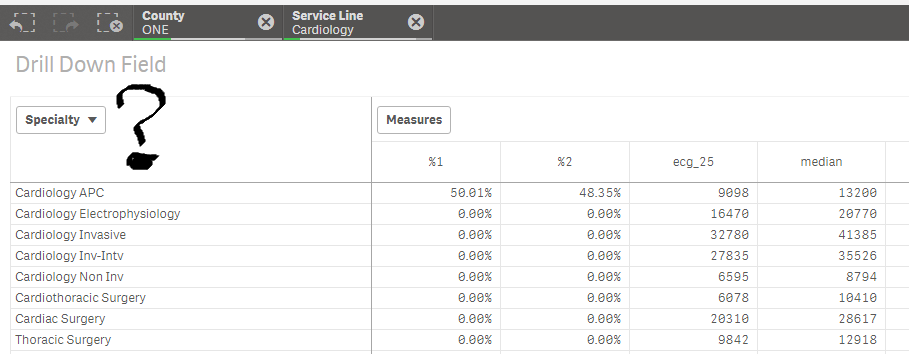# App Development

Announcements
Qlik Insider: SaaS capabilities for Data Integration and Analytics, May 25th! Register Now
cancel
Showing results for
Did you mean:Specialist

## Drill down (level) on a pivot table

Hi community,

I'm using a drill down field on a pivot table.Do you know how can I get the name of the field (level) currently being used on the pivot table?

In the above, if nothing is selected then County will be level being displayed... If only one County is selected and nothing else on the levels, then [Service Line] is supposed to be displayed... and so on. On the image above I'll need to know that "Specialty" is the field being used.

Any ideas? I thought in starting a big IF sentence to go over all levels and confirming if only one value of each is selected but I'm hoping there is a cleverer solution!

Cheers,

Luis

1 Solution

Accepted SolutionsSpecialist
Author

So far this seems to show what the field being used should be:

IF (((GetSelectedCount(County)=1 OR GetPossibleCount(County)= 1) AND (GetSelectedCount([Service Line])=1 OR GetPossibleCount([Service Line])= 1) AND (GetSelectedCount(Specialty)=1 OR GetPossibleCount(Specialty)= 1) AND (GetPossibleCount([MD/DO vs APC])=1 OR GetSelectedCount([MD/DO vs APC])=1)),'PPEET',

IF (((GetSelectedCount(County)=1 OR GetPossibleCount(County)= 1) AND (GetSelectedCount([Service Line])=1 OR GetPossibleCount([Service Line])= 1) AND (GetSelectedCount(Specialty)=1 OR GetPossibleCount(Specialty)= 1)),'[MD/DO vs APC]',

IF (((GetSelectedCount(County)=1 OR GetPossibleCount(County)= 1) AND (GetSelectedCount([Service Line])=1 OR GetPossibleCount([Service Line])= 1)),'Specialty',

IF (((GetSelectedCount(County)=1 OR GetPossibleCount(County)= 1)),'[Service Line]','County'))))

I hope there was something more elegant...

Cheers,

LuisSpecialist
Author

So far this seems to show what the field being used should be:

IF (((GetSelectedCount(County)=1 OR GetPossibleCount(County)= 1) AND (GetSelectedCount([Service Line])=1 OR GetPossibleCount([Service Line])= 1) AND (GetSelectedCount(Specialty)=1 OR GetPossibleCount(Specialty)= 1) AND (GetPossibleCount([MD/DO vs APC])=1 OR GetSelectedCount([MD/DO vs APC])=1)),'PPEET',

IF (((GetSelectedCount(County)=1 OR GetPossibleCount(County)= 1) AND (GetSelectedCount([Service Line])=1 OR GetPossibleCount([Service Line])= 1) AND (GetSelectedCount(Specialty)=1 OR GetPossibleCount(Specialty)= 1)),'[MD/DO vs APC]',

IF (((GetSelectedCount(County)=1 OR GetPossibleCount(County)= 1) AND (GetSelectedCount([Service Line])=1 OR GetPossibleCount([Service Line])= 1)),'Specialty',

IF (((GetSelectedCount(County)=1 OR GetPossibleCount(County)= 1)),'[Service Line]','County'))))

I hope there was something more elegant...

Cheers,

Luis